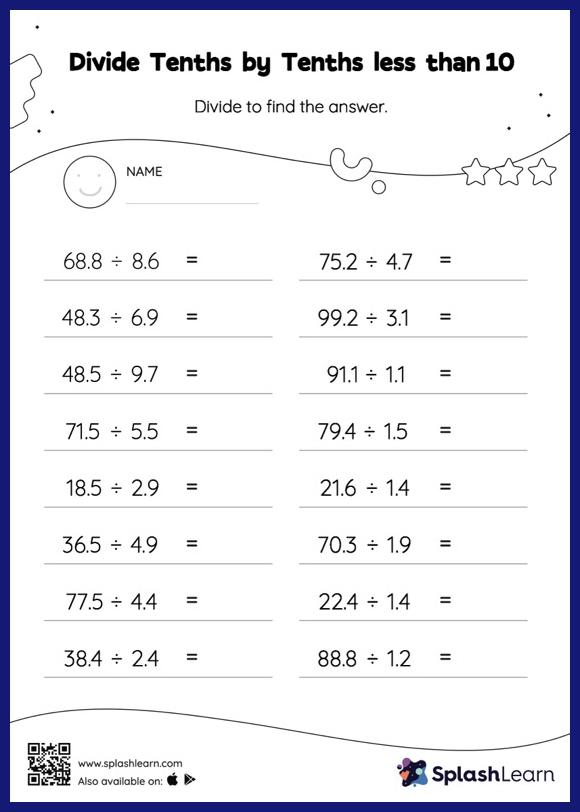# Divide Tenths by Tenths less than 10: Horizontal Division Worksheet

Home > Divide Tenths by Tenths less than 10: Horizontal DivisionThis worksheet challenges young mathematicians to hone their math skills by solving a set of problems on dividing tenths by tenths less than 10. When students divide two decimals, they first divide as if there is no decimal. Depending on the number of decimal digits in each number, they add a decimal point at the appropriate location in the quotient. Students practice this concept in great detail with this divide tenths by tenths less than 10 worksheet.